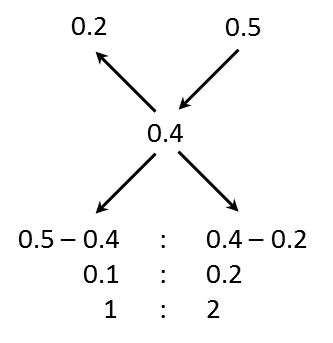# GMAT Quant Practice | Ratio Mixtures Q4

###### GMAT Sample Questions | Mixtures Alligation Question BankGMAT Sample Questions

The given question is a GMAT 600 level problem solving question from Mixtures & Alligation. This GMAT sample question tests the concept of computing the ratio in which two liquids should be mixed. An ideal question to apply the Rule of Alligation to compute the ratio in which two individual components of a mixture should be mixed.

Question 4: In what ratio should a 20% methyl alcohol solution be mixed with a 50% methyl alcohol solution so that the resultant solution has 40% methyl alcohol in it?

1. 1 : 2
2. 2 : 1
3. 1 : 3
4. 3 : 1
5. 2 : 3

## GMAT Live Online Classes

### Explanatory Answer | GMAT Mixtures Alligation Q4

#### Method 1: Using Linear Equations

Let x litres of 20% methyl alcohol solution be mixed with y litres 50% methyl alcohol solution.
If we find the values of x and y, we have found the ratio in which the two liquids are mixed.

Volume Volume of Methyl Alcohol
20% Solution x litres 0.2x
50% Solution y litres 0.5y
Final mixture x + y litres 0.4(x + y)

The volume of methyl alcohol found in the final mixture is the sum of the volumes of methyl alcohol in the 20% and 50% solutions.
0.4x + 0.4y = 0.2x + 0.5y
Or 0.2x = 0.1y
Or $$frac {x} {y}$ = $\frac {0.1} {0.2}$ So, the ratio x : y = 1 : 2 #### Method 2: Using Alligations##### Choice A is the correct answer. ###### GMAT Online Course - QuantTry it free! Register in 2 easy steps and Start learning in 5 minutes! ###### Already have an Account? ###### GMAT Live Online Classes Next Batch Dec 3, 2020 ###### GMAT Coaching in Chennai Next Integrated$Online + Offline) Batch
Starts Thu, Dec 3, 2020

###### GMAT Coaching in Chennai

Sign up for a Free Demo of our GMAT Classes in Chennai

## GMAT Sample Questions | Topicwise GMAT Questions

##### Where is Wizako located?

Wizako - GMAT, GRE, SAT Prep
An Ascent Education Initiative
48/1 Ramagiri Nagar